Courses

# Notes | EduRev

## Class 10 : Notes | EduRev

The document Notes | EduRev is a part of the Class 10 Course Class 10 Mathematics by VP Classes.
All you need of Class 10 at this link: Class 10

[Unless stated otherwise, take π = 22/7]
Q 1. A solid is in the shape of a cone standing on a hemisphere with both their radii being equal to 1 cm and the height of the cone is equal to its radius. Find the volume of the solid in terms of π.
Sol. Here, r = 1 cm and h = 1 cm.
∵ Volume of the conical part = 1/3 πr2h
Volume of the hemispherical part =2/3 πr3
∴ Volume of the solid shape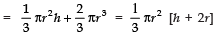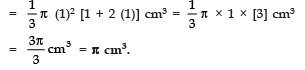Q 2. Rachel, an engineering student, was asked to make a model shaped like a cylinder with two cones attached at its two ends by using a thin aluminium sheet. The diameter of the model is 3 cm and its length is 12 cm. If each cone has a height of 2 cm, find the volume of air contained in the model that Rachel made. (Assume the outer and inner dimensions of the model to be nearly the same.)
Sol. Here, diameter = 3 cm
⇒ Radius (r)= 3/2 cm
Total height = 12 cm
Height of a cone (h1) = 2 cm
∴ Height of both cones = 2 × 2 = 4 cm
⇒ Height of the cylinder (h2) = (12 − 4) cm = 8 cm.
Now, volume of the cylindrical part = πr2h2
Volume of both conical parts =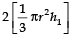∴ Volume of the whole model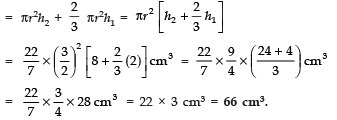Q 3. A gulab jamun contains sugar syrup up to about 30% of its volume. Find approximately how much syrup would be found in 45 gulab jamuns, each shaped like a cylinder with two hemispherical ends with length 5 cm and diameter 2.8 cm (see figure).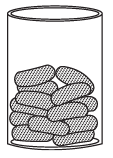Sol. Since a gulab jamun is like a cylinder with hemispherical ends.
Total height of the gulab jamun = 5 cm.
Diameter = 2.8 cm
⇒ Radius = 1.4 cm
∴ Length (height) of the cylindrical part = 5 cm − (1.4 + 1.4) cm
= 5 cm − 2.8 cm = 2.2 cm
Now, volume of the cylindrical part
= πr2h
Volume of a hemispherical end = 2/3 πr3
Volume of both the hemispherical ends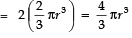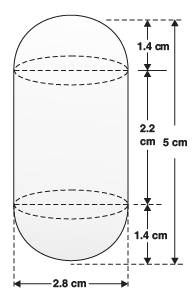∴ Volume of a gulab jamun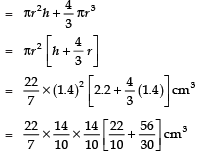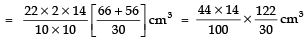⇒ Volume of 45 gulab jamuns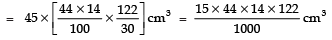Since the quantity of syrup in gulab jamuns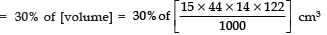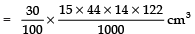= 338.184 cm3
= 338 cm3 (approx.)

Q 4. A pen stand made of wood is in the shape of a cuboid with four conical depressions to hold pens. The dimensions of the cuboid are 15 cm by 10 cm by 3.5 cm. The radius of each of the depressions is 0.5 cm and the depth is 1.4 cm. Find the volume of wood in the entire stand (see Fig.).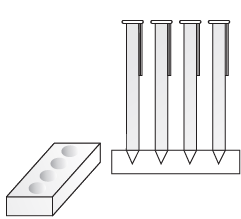Sol. Dimensions of the cuboid are 15 cm, 10 cm and 3.5 cm.
∴ Volume of the cuboid =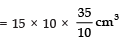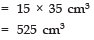Since each depression is conical with base radius (r) = 0.5 cm and depth (h) = 1.4 cm,
∴ Volume of each depression (cone)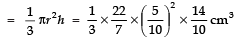Since there are 4 depressions,
∴ Total volume of 4 depressions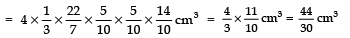Now, volume of the wood in entire stand
= [Volume of the wooden cuboid] − [Volume of 4 depressions]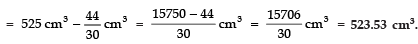Q 5. A vessel is in the form of an inverted cone. Its height is 8 cm and the radius of its top, which is open, is 5 cm. It is filled with water up to the brim. When lead shots, each of which is a sphere of radius 0.5 cm are dropped into the vessel, one-fourth of the water flows out. Find the number of lead shots dropped in the vessel.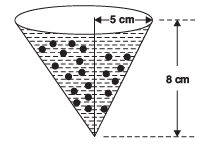Sol. Height of the conical vessel (h) = 8 cm
Base radius (r) = 5 cm
∴ Volume of the cone = 1/3 πr2h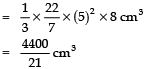Since Volume of the cone = [Volume of water in the cone]
∴ [Volume of water in the cone] =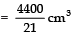Now, Total volume of lead shots = 1/4 of [Volume of water in the cone]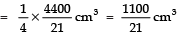Since, radius of a lead shot (sphere) (r) = 0.5 cm
∴ Volume of 1 lead shot =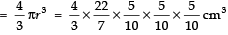∴ Number of lead shots =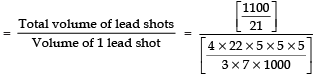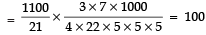Thus, the required number of lead shots = 100.

Q 6. A solid iron pole consists of a cylinder of height 220 cm and base diameter 24 cm, which is surmounted by another cylinder of height 60 cm and radius 8 cm. Find the mass of the pole, given that 1 cm3 of iron has approximately 8 g mass. (Use π = 3.14)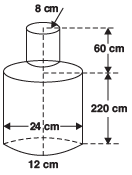Sol. Height of the big cylinder (h) = 220 cm
Base radius (r) = 24/2 cm = = 12 cm
∴ Volume of the big cylinder = πr2h = π (12)2 × 220 cm3
Also, height of smaller cylinder (h1) = 60 cm
Base radius (r1) = 8 cm
∴ Volume of the smaller cylinder πr12h1 = π (8)2 × 60 cm3
∴ Volume of iron
= [Volume of big cylinder] + [Volume of the smaller cylinder]
= π × 220 × 122 + π × 60 × 82 cm3
= 3.14 [220 × 12 × 12 + 60 × 8 × 8] cm3 = 314/100 [20 × 144 + 60 × 64] cm3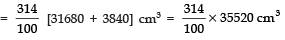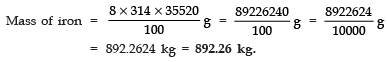Q 7. A solid consisting of a right circular cone of height 120 cm and radius 60 cm standing on a hemisphere of radius 60 cm is placed upright in a right circular cylinder full of water such that it touches the bottom. Find the volume of water left in the cylinder, if the radius of the cylinder is 60 cm and its height is 180 cm.
Sol. Height of the conical part = 120 cm.
Base radius of the conical part = 60 cm.
∴ Volume of the conical part =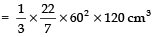Radius of the hemispherical part = 60 cm.
∴ Volume of the hemispherical part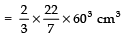∴ Volume of the solid
= [Volume of conical part] + [Volume of hemispherical part]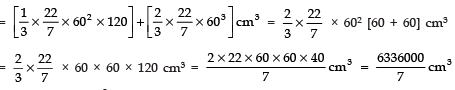Volume of the cylinder = πr2h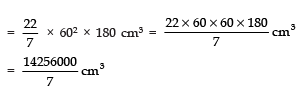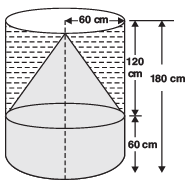⇒ Volume of water in the cylinder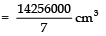∴ Volume of the water left in the cylinder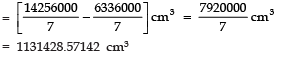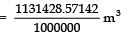[∵ 1000000 cm3 = 1 m3]
= 1.13142857142 m3 = 1.131 m3 (approx).

Q 8. A spherical glass vessel has a cylindrical neck 8 cm long, 2 cm in diameter; the diameter of the spherical part is 8.5 cm. By measuring the amount of water it holds, a child finds its volume to be 345 cm3. Check whether she is correct, taking the above as the inside measurements, and π = 3.14.
Sol. Volume of the cylindrical part
= πr2h = 3.14 × 12 × 8 cm3    [∵ Radius = 2/3 = 1 cm, height (h) = 8 cm]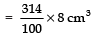Volume of the spherical part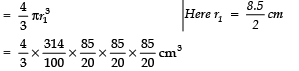Total volume of the glass-vessel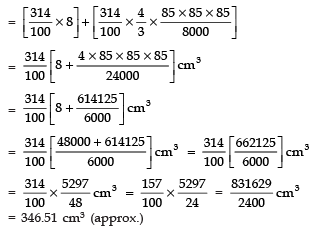⇒ Volume of water in the vessel = 346.51 cm3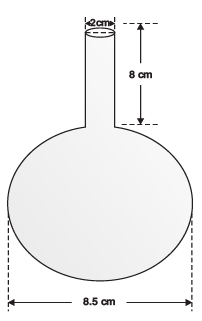Since the child finds the volume as 345 cm3
∴ The child’s answer is not correct
⇒ The correct answer is 346.51 cm3.

Offer running on EduRev: Apply code STAYHOME200 to get INR 200 off on our premium plan EduRev Infinity!

132 docs

,

,

,

,

,

,

,

,

,

,

,

,

,

,

,

,

,

,

,

,

,

;